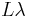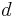# Difference between revisions of "IKONOS"

IKONOS is a commercial earth observation satellite. Details about the sensor are provided at Digital Globe's IKONOS Data Sheet

## Pre-Processing Overview

Typically, multispectral satellite data are converted into physical quantities such as Radiance or Reflectance before they are subjected in multispectral analysis techniques (image interpretation, band arithmetic, vegetation indices, matrix transformations, etc.). The latter can be differentiated in Top of Atmosphere Reflectance (ToAR) which does not account for atmospheric effects (absorption or scattering) and in Top of Canopy Reflectance (ToCR) which introduces a "correction" for atmospheric effects.

In order to derive Reflectance values, likewise as with remotely sensed data acquired by other sensors, IKONOS raw image digital numbers (DNs) need to be converted to at-sensor spectral Radiance values. At-sensor spectral Radiance values are an important input for the equation to derive Reflectance values. Note, Spectal Radiance is the measure of the quantity of radiation that hits the sensor and typically expressed in Failed to parse (lexing error): W * sr^−1 * m^−2 * nm^−1 , that is watts per unit source area, per unit solid angle, and per unit wavelength.

Converting DNs to at-sensor Radiance can be done by using the following equation:$L\lambda = \frac{10^4 * DN\lambda}{CalCoef\lambda * Bandwidth\lambda}$

Converting to Top of Atmosphere Reflectance, also referred to as Planetary Reflectance, can be done by using the following equation:

$\pi * L\lambda * d^2}{ESUN\lambda * cos(θ_S)$

where:

•$\rho$ - Unitless Planetary Reflectance
•$\pi$ - mathematical constant (3.14159265358)
•$L\lambda$ spectral Radiance at the sensor's aperture, from equation... ToADD
•$d$ - Earth-Sun distance in astronomical units, interpolated values
•$Esun$ - Mean solar exoatmospheric irradiance(s) (W/m2/μm), interpolated values
• Failed to parse (lexing error): cos(θ_s) - Solar zenith angle, from the image acquisition's metadata

## Pre-Processing

### Calculate Planetary Reflectance

Converting a Blue Band (Digital Numbers) in to Spectral at-sensor Radiance:

r.mapcalc "Blue_Radiance = ( (10000 * IKONOS_Blue_Band_DN) / (728 * 71.3) )"


Converting the in-Blue spectral band at-sensor Radiance in to Planerary Reflectance:

r.mapcalc "Blue_Reflectance = ( ${PI} * Blue_Radiance *${ESD}^2 ) / ( ${!BAND_Esun} * cos(${SZA}) )"